﻿ 基于LabVIEW的小加速度大脉宽冲击试验台设计

# 基于LabVIEW的小加速度大脉宽冲击试验台设计Design of Small Acceleration and Large Pulse Width Impact Testing Machine Based on LabVIEW

Abstract: With the development of Aeronautics and Astronautics, the requirement of shock resistance for spacecraft and space lifesaving equipment is also increasing. A kind of impact testing machine with small acceleration and large pulse width of 5 - 15 g/100ms is designed. It can simulate the falling impact condition of space equipment better. In this paper, using the PCI-6521 data acquisition card to connect control system and data acquisition system to the computer, in order to remove the noise, acceleration sensor signal conditioning circuit is designed, and the zero drift and filter module are designed in LabVIEW data acquisition. The impact testing machine has been designed and completed. The test system can reliably control the test machine, the small acceleration, large pulse width and half sine shock wave can be generated, and the shock response spectrum can be generated accurately, the shock resistance of the object is analyzed.

1. 引言

2. 系统总体设计

3. 系统硬件设计

3.1. 电荷型加速度传感器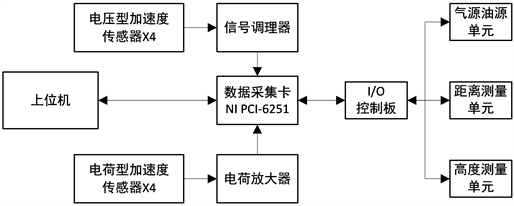Figure 1. System overall block diagram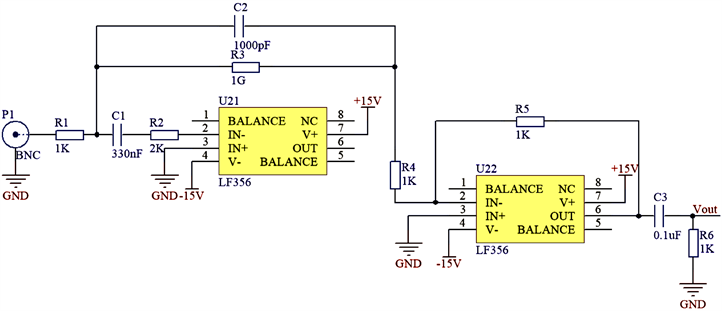Figure 2. Charge amplifying circuit

3.2. 电压型加速度传感器

$I=10\text{μA}\cdot \frac{{R}_{SET}}{{R}_{OUT}}$ (1)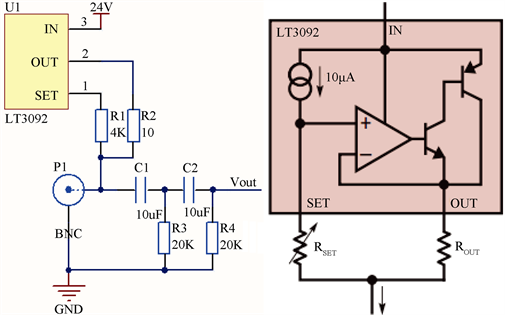Figure 3. IEPE signal conditioning circuit

3.3. 控制系统电路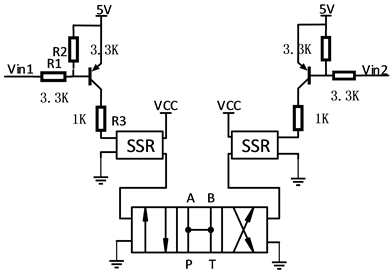Figure 4. Solenoid valve control circuitFigure 5. Proximity switch circuit

4. LabVIEW上位机模块

4.1. 上位机数据采集分析

4.1.1. 容差曲线

4.1.2. 冲击响应谱分析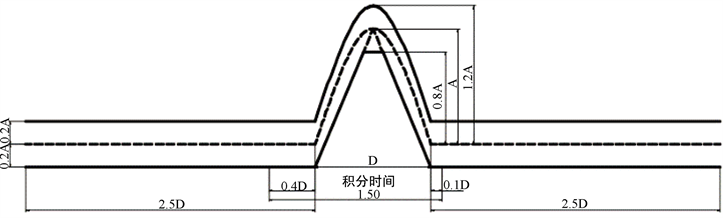Figure 6. National standard half sine wave tolerance curve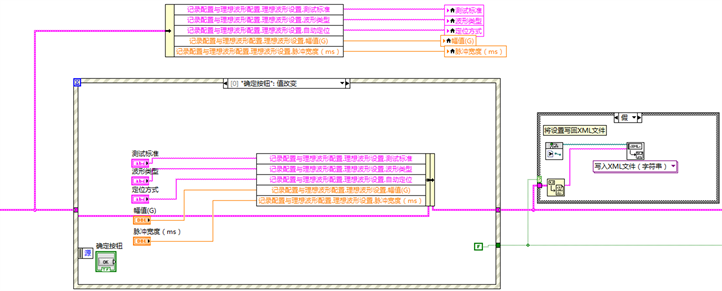Figure 7. Block diagram of tolerance curve setting

${x}_{i}={P}_{0}{U}_{i}+{P}_{1}{U}_{i-1}+{P}_{2}{U}_{i-2}+{q}_{q}{x}_{i-1}+{q}_{2}{x}_{i-2}$ (2)

$\begin{array}{l}{q}_{1}=2{\mathrm{exp}}^{-{\omega }_{n}\zeta }\mathrm{cos}\left({\omega }_{n}\Delta t\sqrt{1-{\zeta }^{2}}\right)\\ {q}_{2}=-{\mathrm{exp}}^{-2{\omega }_{n}\Delta t\zeta }\end{array}$ (3)

$\begin{array}{l}{P}_{0}=\frac{1}{{\omega }_{n}\Delta t}\left[2\zeta \left(\mathrm{exp}\left(-{\omega }_{n}\Delta t\zeta \right)\mathrm{cos}\left({\omega }_{n}\Delta t\sqrt{1-{\zeta }^{2}}\right)-1\right)-E+{\omega }_{n}\Delta t\right]\\ {P}_{1}=\frac{1}{{\omega }_{n}{}^{3}\Delta t}\left[E-{\omega }_{n}\Delta t\mathrm{exp}\left(-{\omega }_{n}\Delta t\sqrt{1-{\zeta }^{2}}\right)+\zeta \left(1+{q}_{2}\right)\right]\end{array}$

$\begin{array}{l}{P}_{2}=-\frac{1}{{\omega }_{n}{}^{3}\Delta t}\left[{q}_{2}\left({\omega }_{n}\Delta t+2\zeta \right)+\zeta {q}_{1}+E\right]\\ E=\left(1-2{\zeta }^{2}\right){\mathrm{exp}}^{-{\omega }_{n}\Delta t}\frac{\mathrm{sin}\left({\omega }_{n}\Delta t\sqrt{1-{\zeta }^{2}}\right)}{\sqrt{1-{\zeta }^{2}}}\end{array}$ (4)

$\begin{array}{l}{x}_{i}={P}_{0}{U}_{i}+{P}_{1}{U}_{i-1}+{P}_{2}{U}_{i-1}+{x}_{i-1}+\left({x}_{i-1}-{x}_{i-2}\right)\\ +\left({q}_{1}-2\right){x}_{i-1}+\left({q}_{2}+2\right){x}_{i-2}\end{array}$ (5)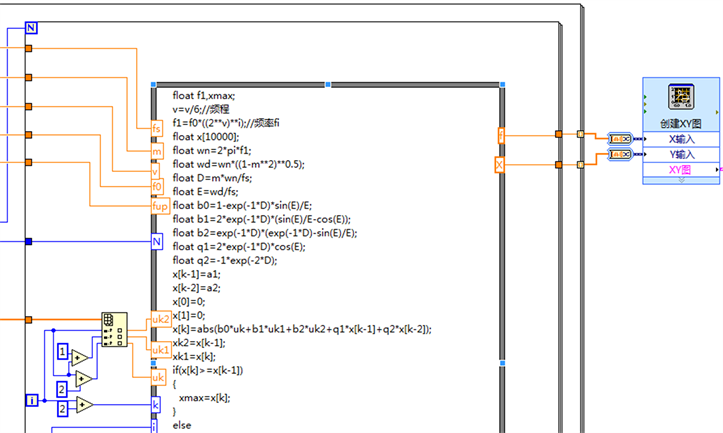Figure 8. Recursive algorithm for shock response spectrum

4.1.3. 设计要点

1) 本系统冲击过程持续时间较短，为了捕捉更加真实的信号需要选用高采样率，但是高采样率也意味着会捕捉到更多的系统高频干扰信号，因此本系统设置采样率为10 KS/s。

2) 由于冲击是一个碰撞过程，在动能转化成势能的过程中，容易产生振动，此外电路和环境中也存在一定的噪声干扰，为提取有用信号，设置了滤波器模块，可根据实际情况选择合适的滤波器和滤波器参数。

4.2. 上位机控制系统设计

5. 实验结果及分析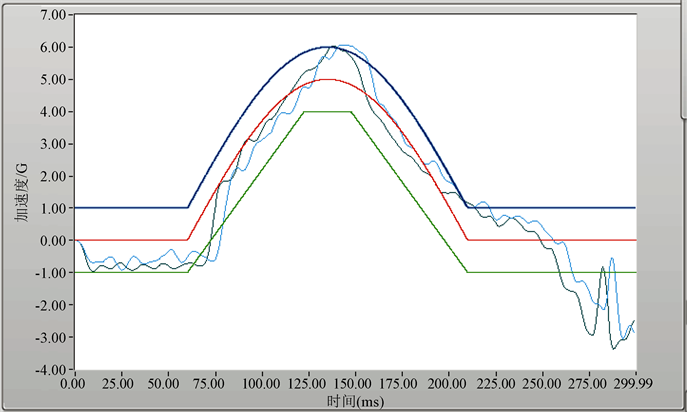Figure 9. Air pressure 0.1 MPa height 500 mm acceleration time curveTable 1. Air pressure 0.1 MPa height 500 mm test resultsTable 2. Air pressure 0.1 MPa height 1000 mm test results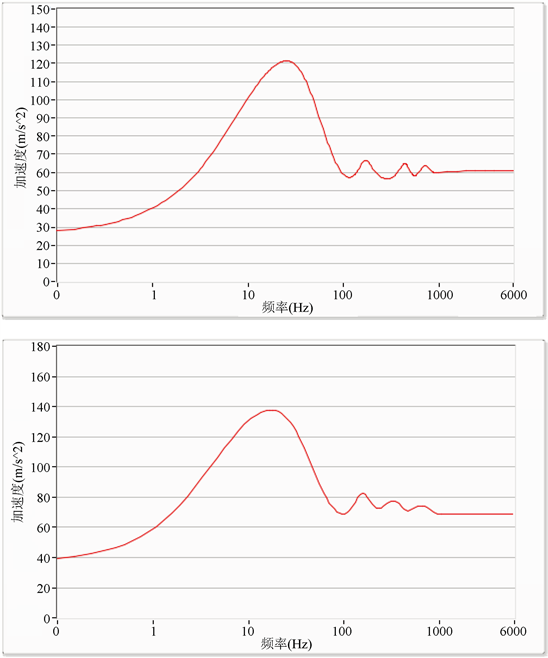Figure 10. Shock response spectrum curveTable 3. Air pressure 0.16 MPa height 2619 mm test results

6. 结论

 胡海岩, 孙久厚, 陈怀海. 机械振动与冲击(修订版) [M]. 北京: 航空工业出版社, 2002.

 Santiago, R., Cantwell, W. and Alves, M. (2017) Impact on Thermoplastic Fibre-Metal Laminates: Experimental Observations. Composite Structures, 159, 800-817.
https://doi.org/10.1016/j.compstruct.2016.10.011

 张荣, 黄含军, 黄海莹, 王松. LabVIEW下冲击试验测试信号分析模块的设计[J]. 计算机测量与控制, 2015(3): 1011-1013, 1037.

 Niskanen, A. and Tuononen, A.J. (2015) Three Three-Axis IEPE Accelerometers on the Inner Liner of a Tire for Finding the Tire-Road Friction Potential Indicators. Sensors, 15, 19251-19263.
https://doi.org/10.3390/s150819251

 李和平. 拉伸、冲击和硬度试验方法国际标准的修订和问题[J]. 理化检验(物理分册), 2010, 46(6): 369-373.

 都军民, 戴宗妙. 冲击响应谱在冲击试验中的应用研究[J]. 舰船科学技术, 2007(S1): 19-21.

 任昌, 潘宏侠. 基于冲击信号的冲击响应谱研究[J]. 火炮发射与控制学报, 2010(3): 21-24.

 邹婿邵. 冲击试验系统控制与数据采集研究[D]: [硕士学位论文]. 长春: 长春理工大学, 2011.

Top